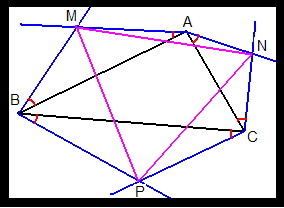All about flooble | fun stuff | Get a free chatterbox | Free JavaScript | Avatarsperplexus dot infoAlways equilateral (Posted on 2008-10-14)Let ABC be any triangle you draw.

From each vertex, draw two lines outside the triangle, each one at 30' (red arcs) with the sides that meet each other in the vertex.

These 6 lines cross, two by two, at 3 points, named M, N, and P.

Prove that, no matter what triangle ABC you draw initially, the triangle MNP is always equilateral.See The Solution Submitted by pcbouhid No RatingComments: ( Back to comment list | You must be logged in to post comments.)Solution| Comment 1 of 5
`Let the letters A, B, C, M, N, and P denote complexnumbers. The givens of the problem imply that`
`   N - A = Z_30*(C - A)`
`   M - B = Z_30*(A - B)`
`   P - C = Z_30*(B - C)`
`where Z_30 = 1/2 + i*sqrt(3)/6.`
`With a lot of tedious math it can be shown that`
`   M - P = Z_60*(N - P)`
`where Z_60 = 1/2 + i*sqrt(3)/2. Therefore, MNP is an equilateral triangle.`
`Note: Multiplying a complex number by         Z_30: rotates it by 30 degrees CCW and            divides its argument by sqrt(3)`
`      Z_60: rotates it by 60 degrees CCW`
` `

 Posted by Bractals on 2008-10-14 15:52:10Please log in:

 Search: Search body:
Forums (0)